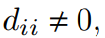# Python code of the Jacobi method in the matrix form

19

## Description

Matrix A can be expressed as:

A = L+ D +U

therefore, the linear system Ax = b can be written as:

(L+ D +U)x = b

The Jacobi method chooses S = D and T = L+U. It is worthy to notice that no diagonal element in D can be 0. That is, for all i = 1,…,n. The Jacobi method is of the form:where,Example :

A = [-5 1 -2; 1 6 3; 2 -1 -4] ;

b = [13; 1; -1] ;

Output :

x =
-2.0000
1.0000
-1.0000

## Reviews

There are no reviews yet.

SKU: 4PYJAHMMUL9 Category: